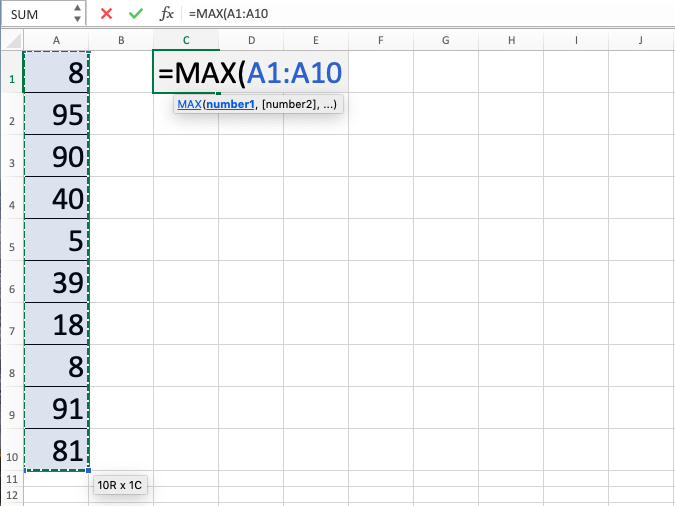MAX Function in Excel - Compute Expert

# MAX Function in Excel

In this tutorial, you will learn about how to use excel MAX function. This formula can be used to find the largest value from a group of numbers that you have in this spreadsheet tool.

## Why We Need to Learn About Excel MAX Function?

In processing number data, often we need to know which is the biggest one in a group of numbers. It is needed, among them, to understand the data most top limit, do analysis based on that biggest digit, or use that knowledge for further data processing.

An example of the largest digit usage in work is when we want to find a product with the highest sales from all products that our company has or know the best quality vendor based on the assessment that the company procurement team does to all vendors who have registered themselves in our system. There are many other cases where we need to know the largest number in a group of data that we have and, of course, it will be much easier if we can do the process automatically in our spreadsheet tool.

In that case, we can use MAX function in excel for the purpose. Excel MAX function can return swiftly the biggest value from all digits which are inputted in this formula writing. This is, of course, in line to answer our needs in the previously described numeric data processing cases.

## What is MAX Function in Excel?

MAX function in excel is a formula with the usage objective to get the largest value in a group of numbers that we have in this excel spreadsheet software.

It is one of the formulas to do ranking processes besides similar kinds of formulas like MIN and RANK. Each has a different usage purpose and can be used according to our needs for number ranking.

A bit of explanation about the inputs in this formula is as follows:

=MAX(number1, number2, …)

Note:
number1, number2, … = all the numbers that you want to find the largest value of

## How to Use Excel MAX Function?

The next part will explain how to use MAX function in excel. In its writing, excel MAX function is pretty easy to understand because it only needs the input of all digits which are wanted to be processed and looked at to find the largest value in them. The input can be in the form of cell range, cell coordinate, or digit.

If you have understood how to use MIN formula previously, then the way to use excel MAX function is quite similar. The difference is only in the objectives, find smallest digit for MIN and the largest one for MAX function in excel.

## Using MAX Function in Excel

1. Type equal sign ( = ) in the cell where you want to put the result in2. Type MAX (can be with large and small letters) and open bracket sign after =3. Drag cursor from the most top left to the most bottom right on the cells where you want to find the largest value. You can also type comma sign ( , ) and add cell range in another place until all of them are in the formula4. Type close bracket sign after all cells that you want to be processed have already been inputted5. Press Enter
6. The process is done!## Exercise

After you have learned about how to use MAX function in excel from the tutorial steps above, you can train your understanding by doing the next exercise!

### Questions

1. What is the largest value in column B?
2. What is the largest value in column C?
3. What is the largest value in column D?

• In using this formula, drag cell cursor and numbers or cell coordinates input with comma sign separation can be done in one writing
• If you want, cell coordinates which are near to each other for excel MAX function input can also be written manually separated for the most top-left cell and most bottom-right cell by two dots sign ( : ) (Writing example: B1:D25)
• There are other formulas to find ranking related data in this spreadsheet software like MIN and RANK. If you want to learn them too, then you can look at the tutorials from other parts of this Compute Expert blog!

Get updated excel info from Compute Expert by registering your email. It's free!